# How to use the causaloptim Shiny app to analyze graphs

library(causaloptim)

Run the Shiny application like so. The result is assigned to an objected called results so that you can save the results that are produced in the web interface.

results <- specify_graph()

A new window in your system’s default web browser is opened with the following interface: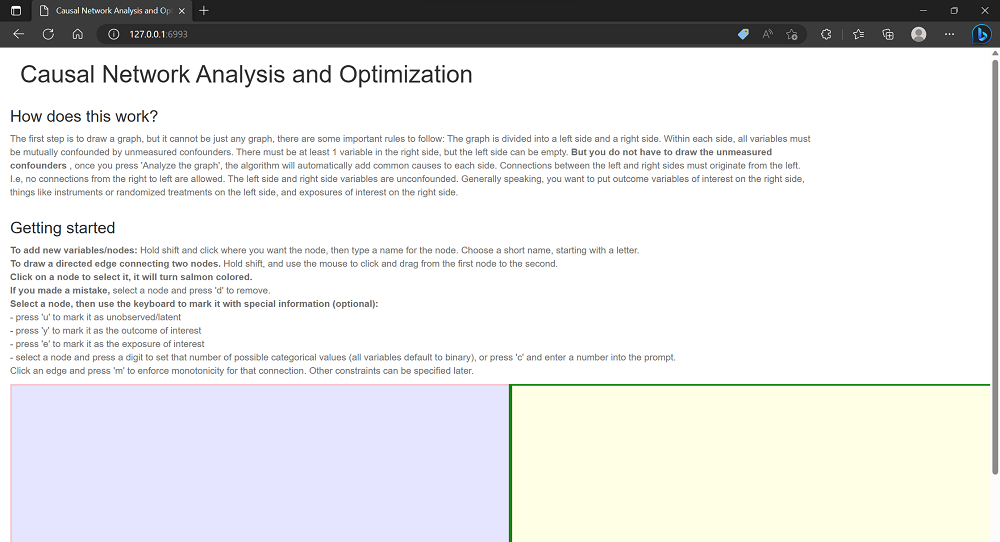Startup

The instructions at the top provide details on how to specify the graph. The graph is divided into a left side and a right side. There is no confounding allowed between the left and right sides. All variables on the right side are confounded. Connections between the left and right sides must originate from the left. I.e, no connections from the right to left are allowed. On the left side, arbitrary connections are allowed and the model assumes that all nodes are observed and connected. On the right side, unmeasured variables are allowed, and the procedure assumes unmeasured confounding between all nodes. These restrictions ensure that the problem can be solved analytically using linear optimization.

In the main window below the text, Shift+click to add nodes. When a node is added, you are prompted to give it a name. Make sure that you use a valid R variable name, but without underscores. This means it cannot start with a number, and cannot contain spaces, underscores or special characters. Single capital letters are good choices, possibly with trailing numbers.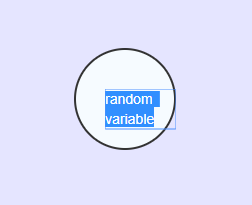Naming nodes

Click on an existing node to select it, and shift+click on a node to change the name. Shift+drag to connect nodes. Remember than no edges may go from the right side to the left side. Click a node to select and then press ‘u’ to mark it as unobserved/latent. Select a node and press a digit to set that number of possible categorical values (all variables default to binary), or press ‘c’ and enter a number into the prompt. Click to select nodes/edges and press ‘d’ to remove. If there are problems selecting nodes, try restarting your browser.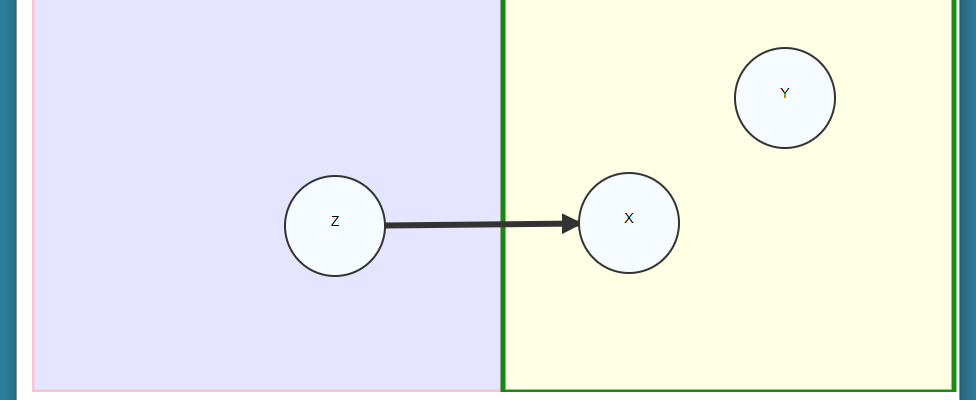Connections

Once we have our graph specified, we can optionally make some annotations. You may specify the outcome of interest, and the exposure of interest. Select the outcome node and press ‘y’ to mark it as the outcome of interest, and then select the exposure node and press ‘e’ to mark it as the exposure of interest. The default causal contrast is the total effect. The exposure will be colored blue, and the outcome colored red.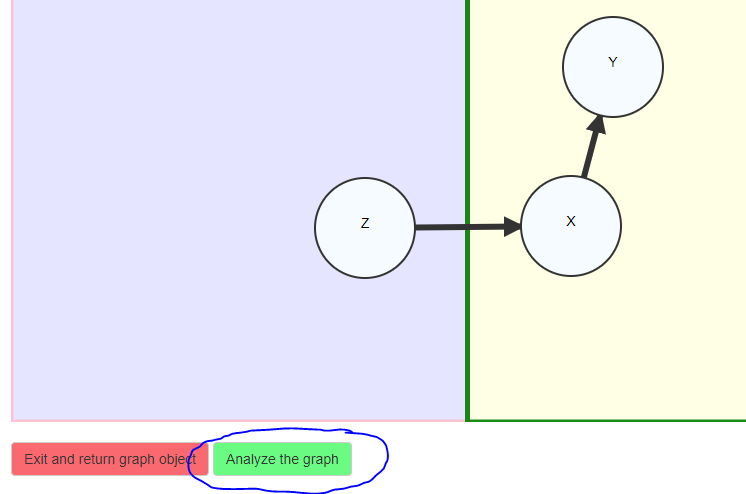Final graph

We now have a graph specified that is ready to be analyzed. Optionally, click an edge and press ‘m’ to enforce monotonicity for that connection. If you are ready to analyze the object click on the “Press to analyze the graph button”. The program interprets the graph, converts it to an igraph object with annotations, and prints the result from R. Inspect the resulting graph carefully so that you understand what is being analyzed. Note that if a side contains at least two variables then a confounder has been automatically added for them.

## Specifying the causal effect of interest

The next step is to use the text box to specify the causal effect of interest. In our current example, we are interested in the causal risk difference in Y comparing X = 1 to X = 0, i.e., Y(X = 1) - Y(X = 0). The optimizer will compute bounds for the expected value of this quantity.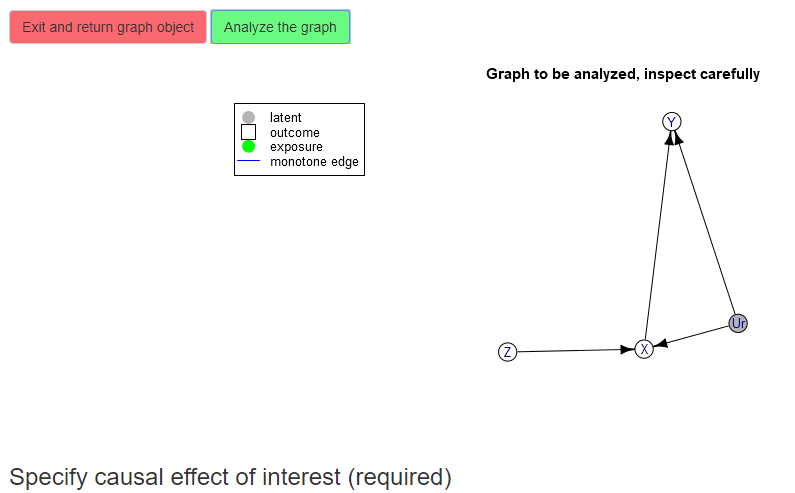Interpreted graph

Use the text box to describe your causal effect of interest. The effects must be of the form

p{V11(X=a)=a; V12(X=a)=b;...} op1 p{V21(X=b)=a; V22(X=c)=b;...} op2 ...

where Vij are names of variables in the graph, a, b are corresponding values, and op are either - or +. You can specify a single probability statement (i.e., no operator). Note that the probability statements begin with little p, and use curly braces, and items inside the probability statements are separated by ;. The variables may be potential outcomes which are denoted by parentheses. Variables may also be nested inside potential outcomes. Pure observations such as p{Y = 1} are not allowed if the left side contains any variables. If the left side contains any variables, then they mush be ancestors of the intervention set variables (or the intervention variables themselves). All of the following are valid effect statements:

p{Y(X = 1) = 1} - p{Y(X = 0) = 1}
p{X(Z = 1) = 1; X(Z = 0) = 0}
p{Y(M(X = 0), X = 1) = 1} - p{Y(M(X = 0), X = 0) = 1}

Back to our example, we specify the effect as

p{Y(X = 1) = 1} - p{Y(X = 0) = 1}

then click the “parse” button to fix the effect.

## Specifying constraints (optional)

Once the causal effect of interest has been specified, you may optionally specify some constraints. Click the button to start the constraint interface.

Here you can specify potential outcomes to constrain by writing the potential outcomes, values of their parents, and operators that determine the constraint (equalities or inequalities). In our example, we can specify monotonicity of the instrument Z by defining the constraint X(Z = 1) $$\geq$$ X(Z = 0):

X(Z = 1) >= X(Z = 0)

then click parse.

## Computation of the bounds

Once you have inspected the graph, specified the causal effect, and understand what is being analyzed, press the final button to compute the bounds.

Note that the computation may take a significant amount of time.

The results are summarized in plain text. The bounds are expressed as the minimum/maximum of a series of expressions involving the conditional probabilities of the observed variables. For more details on the method see this paper. The final button allows you to return all of the objects back to the R environment and store them in results.

The results object contains the graph, the parameterization and interpretation of the graph in terms of the variables and parameters, the bounds and log information about the optimization procedure, and an R function that implements the bounds:

names(results)
#>  "graphres"       "obj"            "bounds.obs"     "constraints"
#>  "effect"         "boundsFunction"

print(results$bounds.obs) #> lower bound = #> MAX { #> p00_0 - p00_1 - p10_1 - p01_1 #> } #> ---------------------------------------- #> upper bound = #> MIN { #> 1 - p10_1 - p01_0 #> } print(results$boundsFunction)
#> function (p00_0 = NULL, p00_1 = NULL, p10_0 = NULL, p10_1 = NULL,
#>     p01_0 = NULL, p01_1 = NULL, p11_0 = NULL, p11_1 = NULL)
#> {
#>     lb <- pmax(p00_0 - p00_1 - p10_1 - p01_1)
#>     ub <- pmin(1 - p10_1 - p01_0)
#>     if (any(ub < lb)) {
#>         warning("Invalid bounds! Data probably does not satisfy the assumptions in the DAG!")
#>     }
#>     data.frame(lower = lb, upper = ub)
#> }

The results object can also be used to numerically simulate the bounds. Try using the simulate_bounds function.

sim <- simulate_bounds(results$obj, results$bounds.obs, nsim = 100)
#>     objective bound.lower bound.upper
#> 1 -0.19499600  -0.4390906   0.2541410
#> 2  0.16548072  -0.3159882   0.4825086
#> 3  0.07622413  -0.4154850   0.2968698
#> 4  0.01544384  -0.3763094   0.3118540
#> 5 -0.24362433  -0.3789019   0.2291424
#> 6  0.17100394  -0.2189083   0.4018218

We also provide a helper function to print the bounds as latex equations. Use the latex_bounds function.

cat(latex_bounds(results$bounds.obs$bounds, results$obj$parameters))

$\mbox{Lower bound} = P(X = 0, Y = 0 | Z = 0) - P(X = 0, Y = 0 | Z = 1) - P(X = 1, Y = 0 | Z = 1) - P(X = 0, Y = 1 | Z = 1)$ $\mbox{Upper bound} = 1 - P(X = 1, Y = 0 | Z = 1) - P(X = 0, Y = 1 | Z = 0)$

Please sends any bugs or feedback to or file an issue on https://github.com/sachsmc/causaloptim .